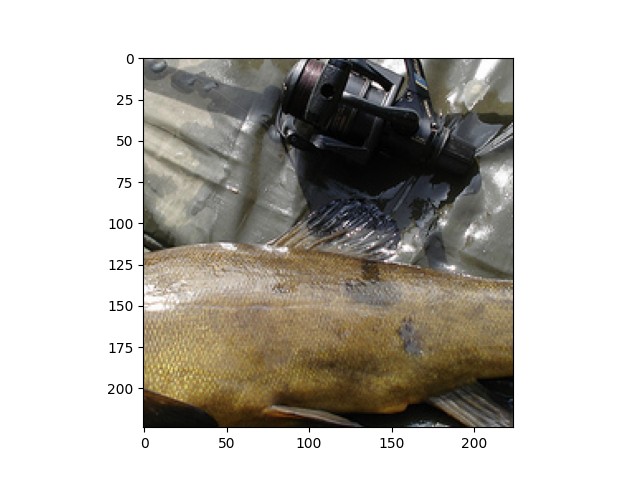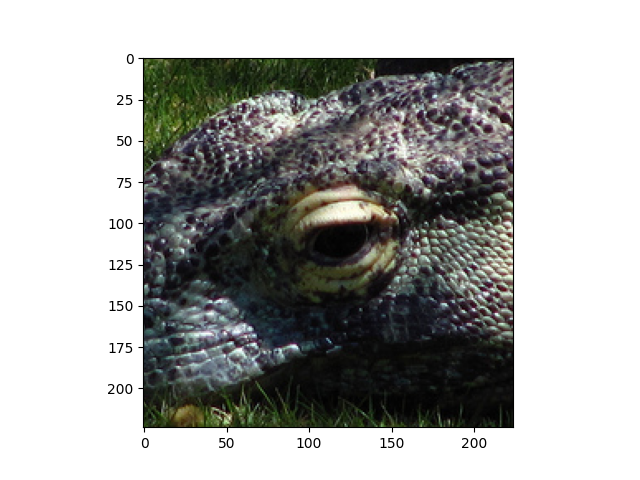# Prepare your dataset in ImageRecord format¶

Raw images are natural data format for computer vision tasks. However, when loading data from image files for training, disk IO might be a bottleneck.

For instance, when training a ResNet50 model with ImageNet on an AWS p3.16xlarge instance, The parallel training on 8 GPUs makes it so fast, with which even reading images from ramdisk can’t catch up.

To boost the performance on top-configured platform, we suggest users to train with MXNet’s ImageRecord format.

## Preparation¶

It is as simple as a few lines of code to create ImageRecord file for your own images.

Assuming we have a folder ./example, in which images are places in different subfolders representing classes:

```./example/class_A/1.jpg
./example/class_A/2.jpg
./example/class_A/3.jpg
./example/class_B/4.jpg
./example/class_B/5.jpg
./example/class_B/6.jpg
./example/class_C/100.jpg
./example/class_C/1024.jpg
./example/class_D/65535.jpg
./example/class_D/0.jpg
...
```

First, we need to generate a .lst file, i.e. a list of these images containing label and filename information.

```python im2rec.py ./example_rec ./example/ --recursive --list --num-thread 8
```

After the execution, you may find a file ./example_rec.lst generated. With this file, the next step is:

```python im2rec.py ./example_rec ./example/ --recursive --pass-through --pack-label --num-thread 8
```

It gives you two more files: example_rec.idx and example_rec.rec. Now, you can use them to train!

For validation set, we usually don’t shuffle the order of images, thus the corresponding command would be

```python im2rec.py ./example_rec_val ./example_val --recursive --list --num-thread 8
python im2rec.py ./example_rec_val ./example_val --recursive --pass-through --pack-label --no-shuffle --num-thread 8
```

## ImageRecord file for ImageNet¶

As mentioned previously, ImageNet training can benefit from the improved IO speed with ImageRecord format.

First, please download the helper script `imagenet.py` validation image info `imagenet_val_maps.pklz`. Make sure to put them in the same directory.

Assuming the tar files are saved in folder `~/ILSVRC2012`. We can use the following command to prepare the dataset automatically.

```python imagenet.py --download-dir ~/ILSVRC2012 --with-rec
```

Note

Extracting the images may take a while. For example, it takes about 30min on an AWS EC2 instance with EBS.

By default `imagenet.py` will extract the images into `~/.mxnet/datasets/imagenet`. You can specify a different target folder by setting `--target-dir`.

The prepared dataset can be loaded with utility class `mxnet.io.ImageRecordIter` directly. Here is an example that randomly reads 128 images each time and performs randomized resizing and cropping.

```import os
from mxnet import nd
from mxnet.io import ImageRecordIter

rec_path = os.path.expanduser('~/.mxnet/datasets/imagenet/rec/')

# You need to specify ``root`` for ImageNet if you extracted the images into
# a different folder
train_data = ImageRecordIter(
path_imgrec = os.path.join(rec_path, 'train.rec'),
path_imgidx = os.path.join(rec_path, 'train.idx'),
data_shape  = (3, 224, 224),
batch_size  = 32,
shuffle     = True
)
```
```for batch in train_data:
print(batch.data.shape, batch.label.shape)
break
```

Out:

```(32, 3, 224, 224) (32,)
```

Plot some validation images

```from gluoncv.utils import viz
val_data = ImageRecordIter(
path_imgrec = os.path.join(rec_path, 'val.rec'),
path_imgidx = os.path.join(rec_path, 'val.idx'),
data_shape  = (3, 224, 224),
batch_size  = 32,
shuffle     = False
)
for batch in val_data:
viz.plot_image(nd.transpose(batch.data, (1, 2, 0)))
viz.plot_image(nd.transpose(batch.data, (1, 2, 0)))
break
```
••Total running time of the script: ( 0 minutes 3.713 seconds)

Gallery generated by Sphinx-Gallery